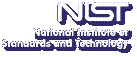UpAn Adaptive Power Method for the Generalized Tensor Eigenproblem

Tamara G. Kolda
Sandia National Laboratories, Livermore

Friday, July 26, 2013 13:00-14:00,
Building 101, Lecture Room D
Gaithersburg
Friday, July 26, 2013 11:00-12:00,
Room 1-4550
Boulder

Abstract:

A tensor is a multidimensional or $m$-way array. Tensor analysis is a higher-order analogue of matrix analysis, and advances in the algorithms for tensor analysis enable researchers in a variety of domains to tackle previously intractable problems. Here we discuss the concept of eigenpairs for tensors and describe a new method for computing them.

Let ${\cal A}$ and ${\cal B}$ be real-valued, symmetric, $m$th-order, $n$-dimensional tensors. Assume further that $m$ is even and ${\cal B}$ is positive definite. We say $(\lambda, x) \in \mathbb{R} \times \mathbb{R}^n - \{0\}$ is a generalized eigenpair (also known as a $\mathcal{B}$-eigenpair) if $${\cal A}x^{m-1} = \lambda{\cal B}x^{m-1} .$$ Here, the tensor-vector product ${\cal A}x^{m-1}$ (or analogously ${\cal B}x^{m-1}$) is defined by $$({\cal A}x^{m-1})_{i_1} = \sum_{i_2}^{n} \cdots \sum^{n}_{i_m=1} a_{i_1 i_2 \cdots i_m} x_{i_2} \cdots x_{i_m} {\rm \ for\ all\ } i_1=1,\cdots,n .$$ This reduces to the matrix generalized eigenproblem for $m = 2$. The advantage of the generalized eigenpair framework is that it nicely encapsulates multiple definitions of tensor eigenvalues, including the $Z$- and $H$-eigenpairs for suitable choices of ${\cal B}$

In this talk, we will describe a method for computing generalized eigenpairs. Our method is a generalization of the shifted symmetric higher-order power method (SS-HOPM) for computing $Z$-eigenvalues; however, we have significantly improved even the original method by adding an adaptive method for choosing the shift. To derive the method, we equate the generalized eigenproblem, to an appropriate nonlinear program such that any generalized eigenpair is equivalent to a KKT point. We develop an adaptive, monotonically convergent, shifted power method for solving the optimization problem (which is potentially useful for finding extrema of any smooth function on the surface of a sphere). We show that our method is much faster than the SS-HOPM method for finding $Z$-eigenpairs due to its adaptive shift selection and also show that it can find other generalized eigenpairs.

This is joint work with Jackson Mayo, Sandia National Laboratories.

Speaker Bio: Tamara (Tammy) Kolda is a Distinguished Member of Technical Staff in the Informatics and Systems Assessments department at Sandia National Laboratories in Livermore, California. Before joining Sandia, she held the Householder Postdoctoral Fellowship in Scientific Computing at Oak Ridge National Laboratory. She received her Ph.D. in applied mathematics from the University of Maryland at College Park. Her research interests include multilinear algebra and tensor decompositions, graph models and algorithms, data mining, optimization, nonlinear solvers, parallel computing, and the design of scientific software. She has received a 2003 Presidential Early Career Award for Scientists and Engineers (PECASE) and a 2008 IEEE International Conference on Data Mining (ICDM08) Best Paper Prize, a 2013 SIAM International Conference on Data Mining (SDM13) Best Paper Prize, and was selected as a 2011 Distinguished Member of the Association for Computing Machinery (ACM). Currently, she serves on the SIAM Board of Trustees, is the Section Editor for the Software and High Performance Computing section of the SIAM Journal on Scientific Computing, is an Editor for the newly formed Journal on Complex Networks (Oxford University Press), and is an Associate Editor for SIAM Journal on Matrix Analysis.

Contact: B. Cloteaux

Note: Visitors from outside NIST must contact Cathy Graham; (301) 975-3800; at least 24 hours in advance.